Introduction to phylogenies in R

## Introduction to phylogenies in R

This tutorial gives a basic introduction to phylogenies in the R language and statistical computing environment.

We can use the package `"ctv"` (i.e., CRAN Task View) to automatically install & update all the packages for R phylogenetic analysis that are available and listed in the Task View.

(Disclaimer - although you can do this on your own, please DO NOT do it during class as it will probably take a while to run & thus will disrupt progress of the activity.)

``````## install CRAN Task View for phylogenetics
## to run, remove the comment character '#'
# install.packages("ctv")
# library("ctv")
# install.views("Phylogenetics")
# update.views("Phylogenetics")
``````

As an alternative to this, you can instead just install a few critical packages, such as ape, geiger, and phytools.

``````## to run, remove the comment character '#'
# install.packages("ape")
# install.packages("geiger")
# install.packages("phytools")
``````

Installing automatically from CRAN using `install.packages` installs not only your target package - but also any libraries on which that package depends.

Since R packages are often an enormous body of work for the developer(s) & maintainer(s), if you use a particular R package for analyses that appear in a publication, it is important that you cite that package - as well as R of course. To find out citation information for a R, simply type:

``````citation()
``````
``````##
## To cite R in publications use:
##
##   R Core Team (2015). R: A language and environment for
##   statistical computing. R Foundation for Statistical Computing,
##   Vienna, Austria. URL http://www.R-project.org/.
##
## A BibTeX entry for LaTeX users is
##
##   @Manual{,
##     title = {R: A Language and Environment for Statistical Computing},
##     author = ,
##     organization = {R Foundation for Statistical Computing},
##     year = {2015},
##     url = {http://www.R-project.org/},
##   }
##
## We have invested a lot of time and effort in creating R, please
## 'citation("pkgname")' for citing R packages.
``````

Similarly, to obtain citation information contributed package that you have downloaded, simply type (for example):

``````citation("geiger")
``````
``````##
## To cite medusa, auteur, or geiger in a publication use:
##
## medusa
##
##   Alfaro Michael E, Francesco Santini, Chad Brock, Hugo Alamillo,
##   Alex Dornburg, Daniel L Rabosky, Giorgio Carnevale, and Luke J
##   Harmon. 2009. Nine exceptional radiations plus high turnover
##   explain species diversity in jawed vertebrates. PNAS
##   106:13410-13414.
##
## auteur
##
##   Eastman Jonathan M, Michael E Alfaro, Paul Joyce, Andrew L Hipp,
##   and Luke J Harmon. 2011. A novel comparative method for
##   identifying shifts in the rate of character evolution on trees.
##   Evolution 65:3578-3589.
##
## MECCA
##
##   Slater Graham J, Luke J Harmon, Daniel Wegmann, Paul Joyce, Liam
##   J Revell, and Michael E Alfaro. 2012. Fitting models of
##   continuous trait evolution to incompletely sampled comparative
##   data using approximate Bayesian computation. Evolution
##   66:752-762.
##
## geiger
##
##   Harmon Luke J, Jason T Weir, Chad D Brock, Richard E Glor, and
##   Wendell Challenger. 2008. GEIGER: investigating evolutionary
``````

Now we’ve installed (either) pretty much every known phylogeny package for R that is available on CRAN - by barely lifting a finger; or some critical packages (ape, phytools, geiger). The most important core package for phylogenies in R is called `"ape"`, which stands for Analysis of Phylogenetics and Evolution in R.

``````## load ape
library(ape)
``````

`"ape"` does many different things. To get started let’s read a ‘toy’ example vertebrate tree from a Newick text string:

``````## read tree from string
tt="(((((((cow, pig),whale),(bat,(lemur,human))),(robin,iguana)),coelacanth),gold_fish),shark);"
``````

We can plot this phylogeny in R in a number of different ways. Let’s plot this phylogeny in three different styles:

``````plot(vert.tree)
``````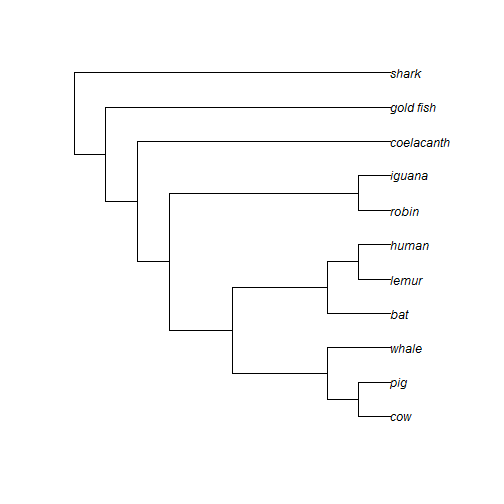``````plot(vert.tree,type="cladogram")
``````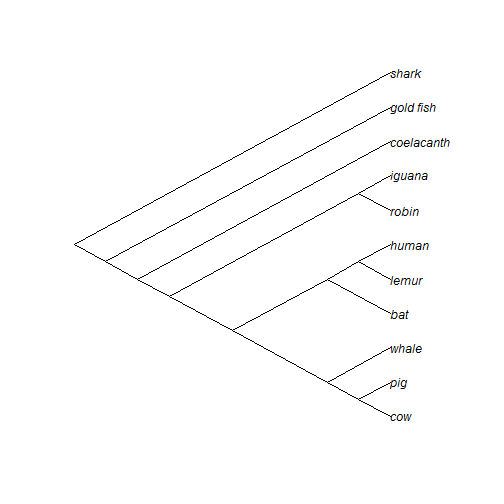``````plot(unroot(vert.tree),type="unrooted")
``````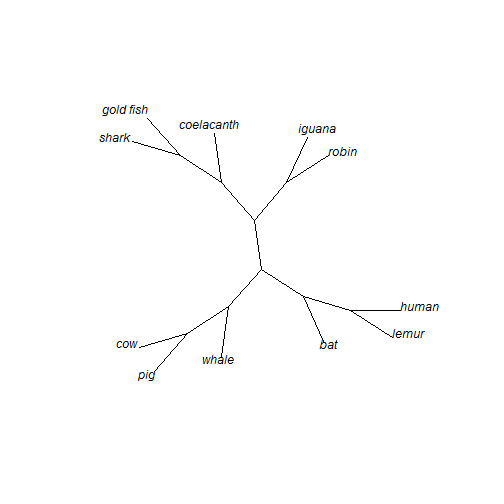``````plot(vert.tree,type="fan")
``````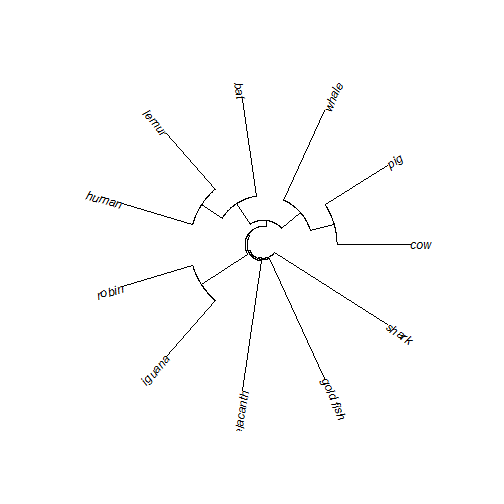The object created in memory when we simulate or estimate a phylogeny, or read one from an input file, is a list of class `"phylo"`. Remember, a list is just a customizable object type that can combine different objects of different types. For instance, a list might have a vector of real numbers (with class `"numeric`) as its first element; and then a vector of strings (with class `"character"`) as its second element; and so on.

An object of class `"phylo"` has at least 4 parts. These are normally hidden, for instance, just typing the name of your `"phylo"` object does not give you the structure in memory, as it does for many R objects:

``````vert.tree
``````
``````##
## Phylogenetic tree with 11 tips and 10 internal nodes.
##
## Tip labels:
##  cow, pig, whale, bat, lemur, human, ...
##
## Rooted; no branch lengths.
``````

However, we can see the structure using:

``````str(vert.tree)
``````
``````## List of 3
##  \$ edge     : int [1:20, 1:2] 12 13 14 15 16 17 18 18 17 16 ...
##  \$ tip.label: chr [1:11] "cow" "pig" "whale" "bat" ...
##  \$ Nnode    : int 10
##  - attr(*, "class")= chr "phylo"
##  - attr(*, "order")= chr "cladewise"
``````

To get at the core of how an object of class `"phylo"` encodes phylogenetic information, let’s use a simple case: a tree with 5 tips & no edge lengths.

``````tree<-read.tree(text="(((A,B),(C,D)),E);")
``````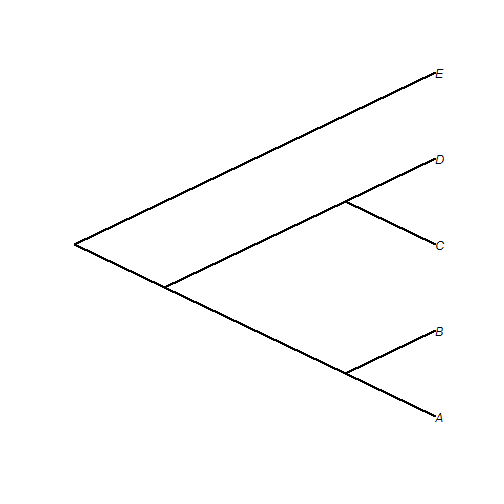The object, in this case, consists of three components and a class attribute:

``````tree\$edge
``````
``````##      [,1] [,2]
## [1,]    6    7
## [2,]    7    8
## [3,]    8    1
## [4,]    8    2
## [5,]    7    9
## [6,]    9    3
## [7,]    9    4
## [8,]    6    5
``````

The matrix `edge` contains the beginning and ending node number for all the nodes and tips in the tree. By convention, the tips of the tree are numbered 1 through n for n tips; and the nodes are numbered n

• 1 through n + m for m nodes. m = n - 1 for a fully bifurcating tree. This is just to keep track of which nodes are internal and which are leaves.
``````tree\$tip.label
``````
``````##  "A" "B" "C" "D" "E"
``````

The vector `tip.label` contains the labels for all the tips in the tree. The order of `tip.label` is the order of the tips numbered 1 through n in `edge`.

``````tree\$Nnode
``````
``````##  4
``````

The integer `Nnode` contains the number of internal nodes in the tree, including the root of the tree if the tree is rooted.

If it seems difficult to imagine that this object could contain all the information in the tree, here is a plotted tree with the edge numbers overlain:

``````plot(tree,edge.width=2,label.offset=0.1,type="cladogram")
nodelabels()
tiplabels()
``````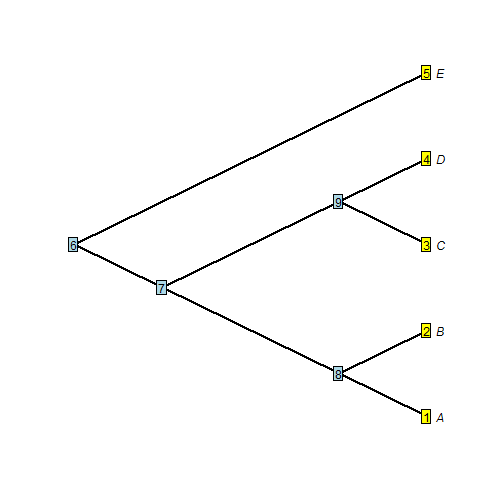We can see that all of the relationship information among the taxa in the tree is containing in the starting & ending nodes for each edge. Edges that share a common starting node number are descended from an immediate common ancestor, etc.

An object of class `"phylo"` also (by definition) has at least one attribute - its class. This is just a value to tell various methods in R what to do with an object of this type. For instance, if we call the method `plot`, R knows to use the method `plot.phylo` in the R package `"ape"`.

`"phylo"` can also have other components, the most common of which are `edge.length` (a vector of class `"numeric"` containing all the edge lengths of the tree in the same order as the rows in `edge`; and `root.edge`, a numeric value giving the length of the root edge, if one exists. In addition, other elements & attributes can be added for special types of phylogenetic trees.

It’s important to keep in mind that this is neither the only way to store a phylogeny in computer memory, nor even the only way to store a tree in R! However the advantage of storing trees in the same way across different packages is that it improves package interoperability - which is a fancy way of saying that developers can concentrate on developing methods that build on existing functionality, rather than reinventing the wheel anew for each project.

For instance, one of many reasons to build on the structures developed for the `"ape"` package, is that there are many different utility functions in `"ape"` and the other R packages for phylogenetics, especially my package `"phytools"`, for reading, writing, and manipulating phylogenetic trees stored in memory using this structure. There are a huge number of “utility” functions of this type for phylogenies, so I’m just going to review some of the more important & useful ones.

### Writing & reading phylogenetic trees

We can easily write & read trees to & from files, for example:

``````write.tree(tree,"example.tre")
``````
``````## (((A,B),(C,D)),E);
``````
``````## load phytools
library(phytools)
writeNexus(tree,"example.nex")
``````
``````## #NEXUS
## [R-package PHYTOOLS, Wed Jul 01 21:19:41 2015]
##
## BEGIN TAXA;
##  DIMENSIONS NTAX = 5;
##  TAXLABELS
##      A
##      B
##      C
##      D
##      E
##  ;
## END;
## BEGIN TREES;
##  TRANSLATE
##      1   A,
##      2   B,
##      3   C,
##      4   D,
##      5   E
##  ;
##  TREE * UNTITLED = [&R] (((1,2),(3,4)),5);
## END;
``````

### Simulating, plotting, extracting clades, & dropping tips

We can also simulate trees, plot them, extract clades, & drop tips from the tree:

``````## (I'm going to first set the seed for repeatability)
set.seed(1)
## simulate a birth-death tree using phytools
tree<-pbtree(b=1,d=0.2,n=40)
## stopping criterion is 40 extant species, in this case
plotTree(tree)
nodelabels()
``````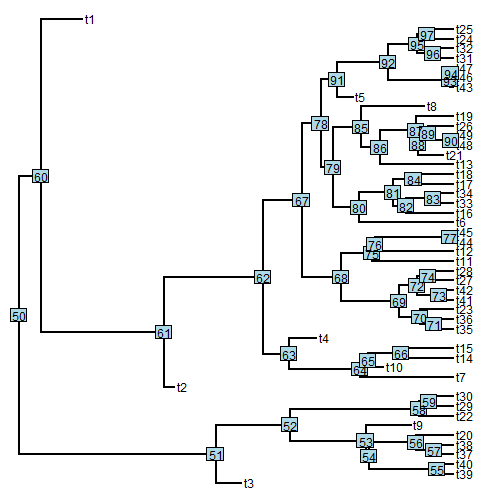``````## ok, now extract the clade descended from node #62
plotTree(tt62)
``````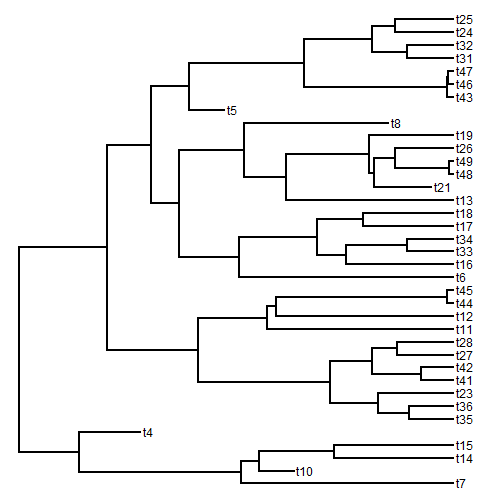``````## now drop 10 tips from the tree (I'm going to pick them at random)
dtips<-sample(tree\$tip.label,10)
dt<-drop.tip(tree,dtips)
plotTree(dt)
``````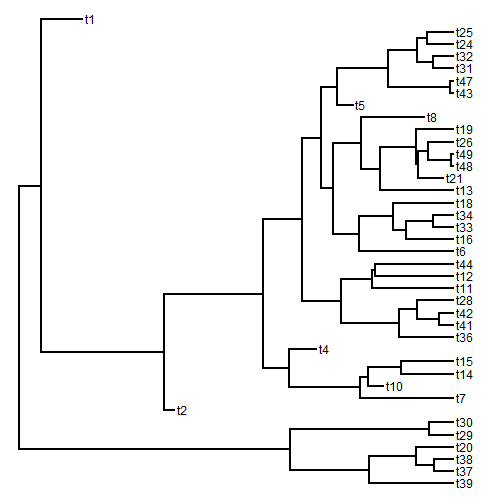``````## we could also, say, drop all tips that go extinct before the present
## this is a fun way, but not the only way to do this:
plotTree(et<-drop.tip(tree,getExtinct(tree)),cex=0.7)
``````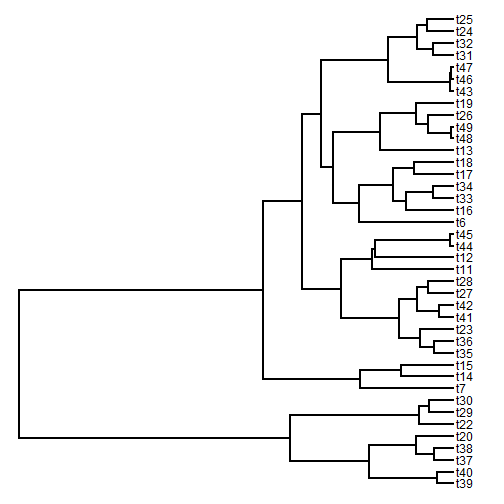### Binary & polytomous trees

`"ape"` and most other phylogenetics packages are equipped to handle phylogenies that are binary or multifurcating; however not all functions will be. We can easily check if our tree is binary, and convert between binary & multifurcating trees.

``````t1<-read.tree(text="((A,B,C),D);")
``````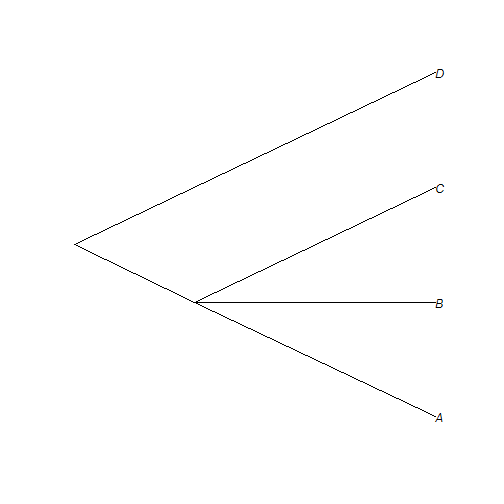``````## check if binary
is.binary.tree(t1)
``````
``````##  FALSE
``````
``````## randomly resolve polytomies
t2<-multi2di(t1)
``````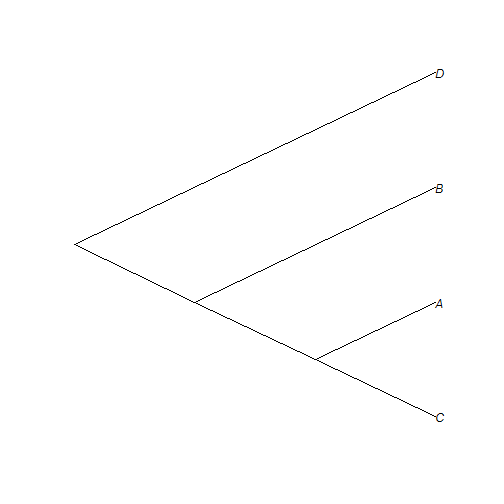``````is.binary.tree(t2)
``````
``````##  TRUE
``````

### Miscellaneous (rotating nodes, re-rooting, etc…)

Lots of other manipulations are possible in R, but here are some simple ones:

``````## rotating nodes
plotTree(tree,node.numbers=T)
``````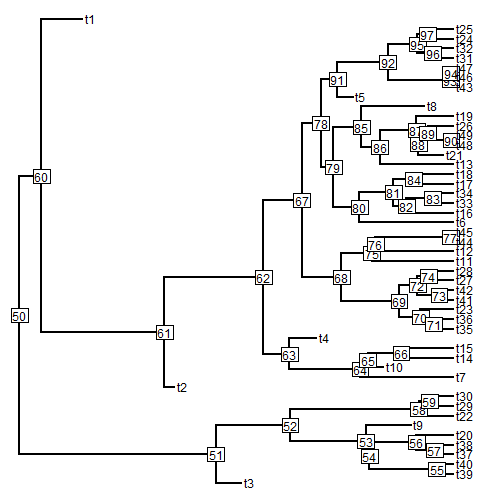``````## first, rotate about node #50
rt.50<-rotate(tree,50)
plotTree(rt.50)
``````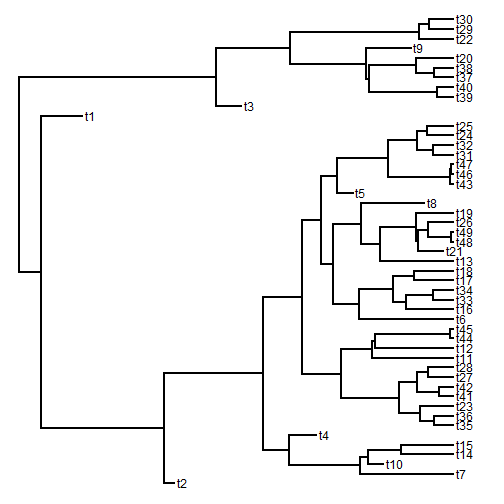``````## now rotate all nodes
rt.all<-rotateNodes(tree,"all")
plotTree(rt.all)
``````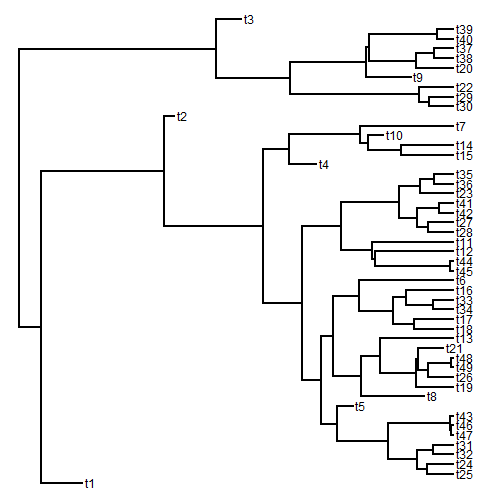``````## ok, now let's re-root the tree at node #67
rr.67<-root(tree,node=67)
plotTree(rr.67)
``````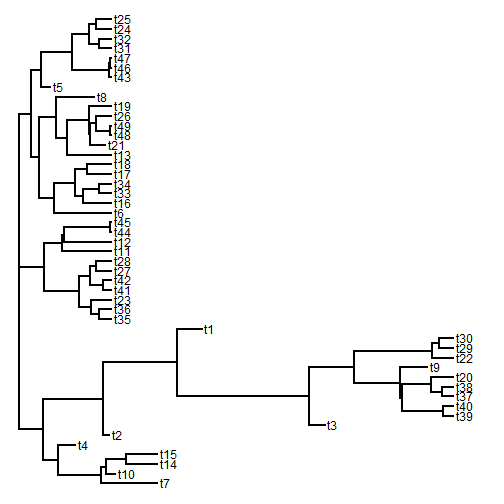``````## this creates a trifurcation at the root
## we could instead re-root at along an edge
rr.62<-reroot(tree,62,position=0.5*tree\$edge.length[which(tree\$edge[,2]==62)])
plotTree(rr.62)
``````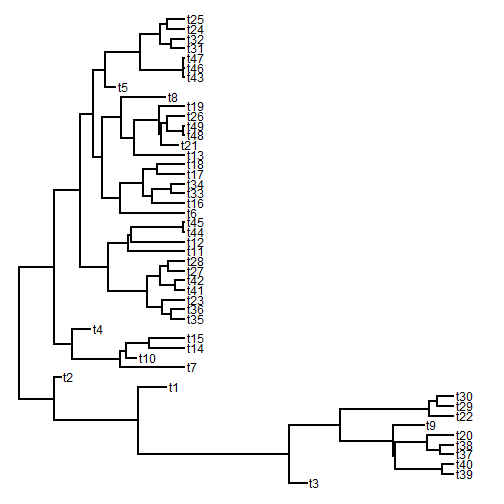### Comparing trees

We can, for example, check to see if our trees are equal. Trees with rotated nodes are equal. Re-rooted trees are not; however they are the same if unrooted. For example:

``````## check if tree & rt.all are equal
all.equal(tree,rt.all)
``````
``````##  TRUE
``````
``````## check if tree & rr.62 are equal
all.equal(tree,rr.62)
``````
``````##  FALSE
``````
``````## check if unrooted tree & rr.62 are equal
all.equal(unroot(tree),unroot(rr.62))
``````
``````##  TRUE
``````

### Multiple trees

Finally, it is sometimes useful to store multiple phylogenies in a single object. This would be the case, for example, if we had a set of trees in a posterior sample from Bayesian phylogeny inference; or if we wanted to replicate a simulation analysis across a large number of phylogenies.

Multiple trees are stored as an object of class “multiPhylo”. This is just a list of objects of class `"phylo"`, with the class attribute `"multiPhylo"`. Many, but not all, functions in `"ape"`, `"phytools"`, and other R packages can be applied to both `"phylo"` and `"multiPhylo"` objects. For instance:

``````## simulate 10 pure-birth trees
trees<-pbtree(n=6,nsim=10,scale=1)
print(trees)
``````
``````## 10 phylogenetic trees
``````
``````## round the edge lengths of the tree to 3 digits
trees<-roundBranches(trees,1)
## write to file
write.tree(trees,file="example.trees")
``````
``````## (t1:1,((t3:0.2,t4:0.2):0.6,(t2:0.7,(t5:0.2,t6:0.2):0.5):0.1):0.2);
## (t1:1,(((t4:0.2,(t5:0.1,t6:0.1):0.1):0,t3:0.2):0.2,t2:0.4):0.6);
## ((t1:0.4,t2:0.4):0.6,(t3:0.3,(t4:0.2,(t5:0,t6:0):0.1):0.2):0.7);
## ((t3:0.2,t4:0.2):0.8,(t1:1,(t2:0.8,(t5:0.1,t6:0.1):0.8):0.2):0);
## ((t3:0.2,t4:0.2):0.8,(((t5:0.1,t6:0.1):0.1,t2:0.3):0.5,t1:0.8):0.2);
## ((t3:0.3,t4:0.3):0.7,((t5:0,t6:0):0.8,(t1:0.5,t2:0.5):0.2):0.2);
## ((t2:0.3,t3:0.3):0.7,(t1:0.3,(t4:0.1,(t5:0,t6:0):0.1):0.2):0.7);
## (((t2:0.4,(t3:0.4,t4:0.4):0.1):0.1,(t5:0.3,t6:0.3):0.3):0.4,t1:1);
## (((t2:0.4,(t3:0.4,t4:0.4):0):0,t1:0.5):0.5,(t5:0.1,t6:0.1):0.9);
## (((t3:0.4,t4:0.4):0.5,t1:0.9):0.1,(t2:0.8,(t5:0.3,t6:0.3):0.5):0.2);
``````

Many other tasks are also possible in R phylogenetics. For an overview, read the CRAN Phylogenetics Task View. Also check out my blog: http://blog.phytools.org, or review the PDF manuals of different R packages such as ape, phytools, phangorn, and geiger.

Written by Liam J. Revell. Last updated July 1, 2015